# Automobile Radiator Integrated with Al2O3 Nanofluid for Compact Size and Sustainability Enhancement

Automobile Radiator Integrated with Al2O3 Nanofluid for Compact Size and Sustainability Enhancement

College of Engineering, West Texas A&M University, Canyon, Texas 79016, USA

Corresponding Author Email:
rissa@wtamu.edu
Page:
1057-1065
|
DOI:
https://doi.org/10.18280/ijht.390403
11 July 2021
|
Accepted:
24 August 2021
|
Published:
31 August 2021
| Citation

OPEN ACCESS

Abstract:

An assessment study was conducted making use of the heat transfer enhancement capability of alumina nanoparticles in reducing the size of an automobile radiator cooling system. Experimental data was compiled from different resources to examine the sensitivity of the thermophysical properties of the bulk fluid to the addition of nanoparticles to the base fluid. Correlations were obtained for a radiator-type heat exchanger. The Nusselt number of the bulk fluid is shown to increase by 50% with the introduction of 1% by volume of alumina nanoparticles to the base fluid. Life cycle assessment was conducted on a conventional-size radiator system for 2017 Subaru Impreza with a 2.0 L engine, and on a resized radiator system having the same effectiveness but using 1% by volume of alumina nanoparticles added to the base fluid. For the same heat exchanger effectiveness, the introduction of 1% by volume of alumina nanoparticles to the base fluid reduces the size of the radiator by 26%, the water pump by 60% and the cooling fan by 28%. An economic input-output life cycle assessment shows a reduction of 28% in the consumed energy and global warming potential, and a reduction of 29% in water usage and total air releases.

Keywords:

alumina, global warming potential, life cycle assessment, nanofluid, radiator heat exchanger

1. Introduction

Over the past several years, intensive efforts have been made to design heat exchangers in a way to maximize their thermal performance and reduce their operating cost. Several investigators have studied the benefits of using nanofluids in shell-and-tube heat exchangers [1-8]. The heat exchangers used in their studies consisted of a single shell with n tubes and one flow pass. The alumina nanoparticle size used ranged from 13 to 80 nm, and its concentration in water ranged from 0.03 to 20% by volume. Farajollahi et al.  and Rao et al.  measured the heat transfer characteristics under turbulent flow conditions, while Shahrul et al.  and Barzegarian et al.  investigated laminar flow conditions, and Akhtari et al.  run experiments under both conditions. Farajollahi et al.  saw an enhancement of 24% in the overall heat transfer coefficient at a nanoparticle concentration of 0.3% by volume using 25 nm g-Al2O3 particles. The overall heat transfer coefficient was also shown to increase with the increase in nanoparticle concentration. Rao et al.  observed more than 200% enhancement in the overall heat transfer rate when using alumina nanoparticles having a mean diameter of 22 nm at 0.53% volume concentration. The authors also observed an increase in the heat transfer rate with the increase in nanoparticles volume concentration. The increase in Reynolds number also led to a decrease in the nanofluid friction factor. Shahrul et al.  saw an enhancement of 15% in the heat transfer coefficient at a nanoparticle volume concentration of 0.5% using 13 nm particles. The authors used ultrasonic vibration to reduce particle agglomeration in the water-based fluid. The heat transfer rate was shown to increase with the volumetric flow rate but reach saturation. Barzegarian et al.  used g-Al2O3 nanoparticles with a mean diameter of 15 nm. A maximum enhancement in the overall heat transfer coefficient of 19.1% was seen at a volume concentration of 0.3%. They attributed the increase in the heat transfer performance to the Brownian motion of the nanoparticles and to the reduction of the boundary layer thickness. Their findings also revealed a minor increase in the friction factor. Akhtari et al.  saw an enhancement of 28.6% in the heat transfer rate compared to pure water when using 80 nm alumina particles at a concentration of 0.5% by volume. Their results indicated that the heat transfer performance of the heat exchanger increased with the increase in nanoparticles concentration and nanoparticles inlet temperature. Computational fluid dynamics (CFD) simulations revealed an average relative error of 15% with their experimental data. Ahmed et al.  conducted experiments to investigate the performance of chilled-water air-conditioning unit using alumina nanoparticles ranging from 20 to 70 nm in size. Nanofluids with nanoparticle concentrations ranging from 0.1 to 1% by weight were used. The coefficient of performance of the cooling unit was seen to increase by 5% and 17% at alumina nanoparticles concentration of 0.1% and 1%, respectively. Anitha et al.  studied the influence of a hybrid Al2O3-Cu nanoparticle concentration on the performance of a single pass shell and tube heat exchanger. A multiphase mixture model using finite volume method was developed to model the flow, and the results were validated with experimental findings. The maximum enhancement in the heat transfer coefficient of the hybrid fluid was found to be 139% in comparison to pure water. Pressure drop was shown to increase with Reynolds number and a significant decrease was observed at the critical point of Reynolds number. Hojatt  developed an artificial neural network to predict the thermal and hydrodynamic behavior of nanofluids in a shell and tube heat exchanger. An artificial neural network was developed by imitating the human nervous system in modeling the non-linear problem of predicting the Nusselt number and pressure drop in a shell and tube heat exchanger from their training values. A multi-layer perceptron artificial neural network was trained according to the thermal and hydrodynamic experimental data the author obtained on TiO2 and Al2O3 nanofluids. Nusselt number values were predicted with an accuracy of 9% and pressure drops were obtained with an accuracy close to 10%.

Peyghambarzadeh et al. , Raei et al. , Issa , and Ramalingam et al.  have experimented with fin-and-tube heat exchangers using 20 and 50 nm alumina nanoparticles with concentrations of up to 1% by volume. Peyghambarzadeh et al.  conducted forced convective heat transfer experiments on an automobile radiator using alumina nanoparticles with concentrations ranging from 0.1 to 1% by volume. Results show that increasing the fluid circulation rate can improve the heat transfer performance with the heat transfer efficiency improving by up to 45% at the highest nanoparticles concentration. However, the fluid inlet temperature to the radiator had insignificant effect. Raei et al.  conducted studies on a fin-and-tube heat exchanger showing improvement of 20% in the overall heat transfer coefficient at 0.2% concentration by weight. This also resulted in 5% increase in pressure drop. It was shown that the presence of a surfactant in the fluid reduces the effect of the nanoparticles in the heat transfer enhancement. Tests conducted by Issa  on the radiator heat exchanger show substantial enhancement in heat exchanger effectiveness of up to 49% at a volume concentration of 0.8%. The results demonstrated that the application of nanofluids in low concentrations was sufficient to cause a significant increase in the radiator thermal performance. Ramalingam et al.  used a hybrid Al2O3-SiC nanofluid in an automotive radiator. Both milled and unmilled SiC nanoparticles were experimented on. Their results showed a thermal enhancement in the Nusselt number of 23.5% when Al2O3 was doped with milled SiC at a volume concentration of 0.8%, while the improvement was 9% for 0.4% volume concentration. The hybrid nanofluid was dispersed in a base fluid at 50:50 volumetric proportions of distilled water and ethylene glycol. Furthermore, milled SiC nanoparticles when added with Al2O3 nanoparticles resulted in higher bulk fluid thermal conductivity and viscosity than when using unmilled SiC nanoparticles.

In his recent review study on alumina nanofluids, the author  found out that the size of the nanoparticle does not have a major influence on the thermal conductivity of the nanofluid. His review showed that published research findings presented contradicting results. Instead of being influenced by the nanoparticle size, thermal conductivity enhancement was shown to depend on the clustering of the nanoparticles in the base fluid. The less compact the structure of the particles clustering is, the greater is the enhancement in the bulk fluid thermal conductivity. The author  also established that increase in nanoparticle concentration leads to an enhancement in the nanofluid Nusselt number and therefore the bulk fluid thermal efficiency. This enhancement is shown to be more significant as the fluid transitions to turbulent flow.

Noticeable enhancement in heat exchangers thermal performance has been achieved at concentrations less than 1% by volume. Because of the ability of nanofluids to increase heat transfer, heat exchangers can be designed to be of smaller size when nanofluids are used instead of conventional fluids. This affects the weight of the equipment and the power consumption of the pumps and fans. The objective of this study is to perform a life cycle assessment study on a nanofluid-integrated automobile radiator to determine its energy savings and carbon footprint reductions.

2. Thermophysical Properties of Al2O3 Nanofluids

Thermophysical properties of water-based alumina nanofluids have been widely investigated by several researchers. This paper compiles the experimental data from different resources to examine the sensitivity of the thermophysical properties to the addition of nanoparticles to the base fluid. Empirical correlations are then obtained. Properties strongly sensitive to the addition of nanoparticles are thermal conductivity, specific heat and viscosity.

2.1 Thermal conductivity

Studies have shown that the thermal conductivity of alumina nanofluids to increase with the increase in the nanoparticles concentration [14-22]. Thermal conductivity compiled from different studies is shown in Figure 1 as function of the nanoparticles volume concentration. Since the majority of the studies in the open literature were conducted on alumina nanofluids using a water-based fluid, the thermal conductivity of alumina nanofluid was normalized to that of pure water in order to obtain a relationship for the enhancement in the bulk fluid thermal conductivity. The size of nanoparticles used in these studies ranges between 5 and 120 nm. It should be noted that the nanoparticle size does not appear to have a major influence on the thermal conductivity enhancement of the nanofluid. These research findings show contradicting results. Issa , shows a decrease in the thermal conductivity of Al2O3 nanofluids with the increase in particle size, while Mintsa et al.  shows an increase. Research findings show no obvious trend to the effect of the nanoparticle size on thermal conductivity.

## 1.pngFigure 1. Thermal conductivity ratio, $\lambda_{\mathrm{nf}} / \lambda_{\mathrm{H} 2 O}$, versus nanoparticle concentration, $\phi_{\mathrm{v}, \mathrm{nf}}$

2.2 Specific heat

Limited experimental studies have been conducted on the specific heat of alumina nanofluids. The normalized data shown in Figure 2 are that of the author . Experimental data show the specific heat to decrease with the increase in particles concentration. In this case as the concentration increases from 0 to 10% by volume, the specific heat decreases by about 25%. Results show specific heat to decrease with the decrease in nanoparticles size.

2.3 Viscosity

Figure 3 shows alumina nanofluid dynamic viscosity data compiled from different studies [14, 16, 19, 23-25]. The data has been normalized with respect to the viscosity of pure water. The figure shows perturbation in the data due to the variation in the alumina nanoparticle size between the studies. Research using very fine particles is scarce except those by Timofeeva et al.  using 11 nm particles showing the bulk fluid to become more viscous with the decrease in the nanoparticle size. At 10% Al2O3 volume concentration, Figure 3 shows the bulk fluid viscosity to more than double.

## 2.png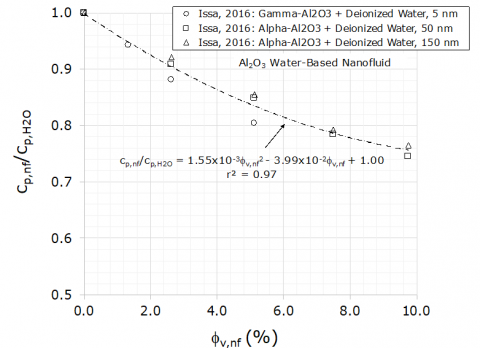Figure 2. Specific heat ratio, cp,nf/cp,H2O, versus nanoparticle concentration, $\phi_{\mathrm{v}, \mathrm{nf}}$

## 3.png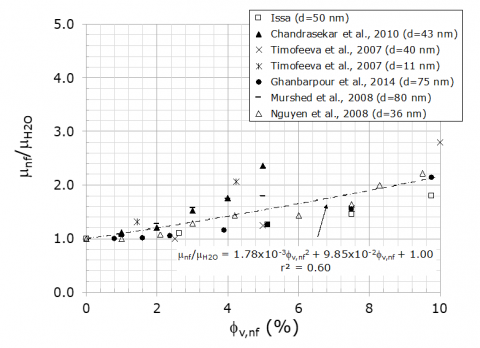Figure 3. Dynamic viscosity ratio, $\mu_{\mathrm{nf}} / \mu_{\mathrm{H} 2 \mathrm{O}}$, versus nanoparticle concentration, $\phi_{\mathrm{v}, \mathrm{nf}}$

3. Performance of Al2O3 Nanofluids in Heat Exchangers

Peyghambarzadeh et al. , Raei et al. , and Issa  have performed heat transfer studies on radiator heat exchangers. The enhancement in Nusselt number is shown as function of the alumina nanoparticle volume concentration (Figure 4). The results show Nusselt number to increase with the increase in the concentration of the alumina nanoparticles. However, it is not clear if the nanoparticle size has an effect on the heat transfer enhancement. Nusselt number is shown to enhance by around 50% at concentration levels of 1% by volume. Wu et al. , Darzi et al. , and Akyurek et al.  conducted studies on heat exchangers where the alumina fluid friction coefficient was experimentally determined. Figure 5 compiles this data showing the normalized nanofluid friction coefficient as function of the nanoparticles concentration. The smaller the particle size, the higher is the friction.

## 4.pngFigure 4. Nusselt number ratio, Nunf/NuH2O, versus nanoparticle concentration, $\phi_{\mathrm{v}, \mathrm{nf}}$

## 5.pngFigure 5. Friction coefficient ratio, fnf/fH2O, versus nanoparticle concentration, $\phi_{\mathrm{v}, \mathrm{nf}}$

4. Sizing of a Nanofluid Integrated Automobile Radiator

Heat transfer enhancement capability of nanofluids is a key factor in helping reduce the size of an automobile radiator system. Through the use of the empirical correlations developed earlier, a nanofluid-integrated automobile radiator can be resized. The effectiveness of a heat exchanger, e, can be expressed as function of the number of transfer units, NTU, the heat capacity rate ratio, Cr, and the flow arrangement in the heat exchanger:

$\varepsilon=f\left(N T U, C_{r}\right.$, Flow Arrangement $)$      (1)

For two automobile radiators of similar geometries with one using a conventional coolant while the other using a nanofluid coolant to have the same thermal effectiveness, the two radiator systems are related through the following equations. Let subscript 1 denote a conventional automobile radiator system, and subscript 2 denote a radiator utilizing a nanofluid coolant. The heat transfer area of system 2 in relation to system 1 is:

$\frac{A_{2}}{A_{1}}=\frac{U_{1}}{U_{2}} \frac{\dot{m}_{a, 2}}{\dot{m}_{a, 1}}$      (2)

U is the radiator overall heat transfer coefficient, and A is the area of interface between the hot and cold sides of the heat exchanger. $\dot{m}_{a}$ is the mass flow rates of air from a blowing fan. The overall heat transfer coefficient ratio is:

$\frac{U_{1}}{U_{2}}=\frac{\frac{1}{h_{f, 2}}+\frac{1}{\eta_{o} h_{a, 2}} \frac{A_{h, 2}}{A_{c, 2}}}{\frac{1}{h_{f, 1}}+\frac{1}{\eta_{o} h_{a, 1}} \frac{A_{h, 1}}{A_{c, 1}}}$      (3)

where, hf is the radiator fluid heat transfer coefficient, ha is the air heat transfer coefficient, ho is overall efficiency of the radiator finned surface at the cold side, Ah is the surface area of the hot side of the radiator, and Ac is the surface area of the cold side of the radiator. The pressure drop in the fluid of radiator 2 in relation to radiator 1 is expressed as follows:

$\left(\frac{\Delta P_{2}}{\Delta P_{1}}\right)_{f} \sim \frac{f_{f, 2}}{f_{f, l}} \frac{L_{f, 2}}{L_{f, 1}}\left(\frac{V_{f, 2}}{V_{f, 1}}\right)^{2}$     (4)

where, $\Delta P_{f}$ is the pressure drop in the fluid flow, ff is the fluid friction coefficient, Vfis the fluid velocity, and Lf is the tubing length. The pressure drop in the air is:

$\left(\frac{\Delta P_{2}}{\Delta P_{1}}\right)_{\text {air }} \sim\left(\frac{V_{a, 2}}{V_{a, 1}}\right)^{1.56} \frac{L_{a, 2}}{L_{a, 1}}\left(\frac{D_{a, 2}}{D_{a, 1}}\right)^{-1.44}$      (5)

where, La and Da are the heat exchanger length and hydraulic diameters associated with the air flow, respectively. Va is the velocity of the air flow. The relationship between system 1 and 2 pump and fan power is:

$\left(\frac{\wp_{2}}{\wp_{1}}\right)_{\text {pump, fan }}=\left(\frac{\Delta P_{2}}{\Delta P_{1}}\right)^{2 / 5}$     (6)

Eq. (2), (4) and (5) are plotted against alumina particle concentration and are used in sizing radiator system 2 for the heat exchanger size, fan and pump pressure drop relative to a conventional radiator system (system 1). The following parameters are used as baseline parameters for radiator system 1 : Ah = 0.59 m2, Ac = 5.29 m2, Af= 4.7 m2, hf = 0.95. Figure 6 shows the sizing of radiator system 2 in relationship to radiator system 1.

## 6.pngFigure 6. Sizing of a nanofluid-integrated radiator system

5. Life Cycle Assessment of a Nanofluid-Integrated Radiator

Life cycle assessment study was conducted on an automobile radiator system using the economic input-output life cycle assessment (EIO-LCA) online model of the 2002 U.S. economy  based on Wassily Leontief’s  input-output breakdown of the economy. In this model, the entire U.S. economy is aggregated into 428 sectors. The model represents the various inputs that are required to produce a unit of output in each economic sector. By assembling all the sectors, the model is able to trace all the direct and indirect inputs to produce outputs in each sector. The EIO-LCA model is a linear model such that an increase in the output of goods and services from any sector will result in a proportional increase in each input received from all the other sectors in the economy. The study was conducted on: 1) a conventional radiator system, and 2) a resized radiator system using alumina nanofluid. Two life cycle phases were examined: 1) the manufacturing and 2) the operation phase (Figure 7).

## 7.png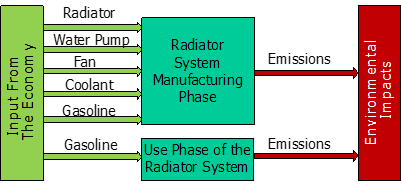Figure 7. Radiator systems manufacturing and use life phases

Table 1. 2017 Subaru Impreza weight and fuel economy data

 Parameter Metrics Test Weight 1,422 kg Fuel Economy City (12x106 m per m3 of fuel), Highway (16x106 m per m3 of fuel) EPA-rated Fuel Economy (55% City, 45% Highway) 14x106 m per m3 of fuel Weight to Fuel Economy Correlation 10% weight reduction = 4.38% fuel consumption reduction

A traditional radiator system consisting of the heat exchanger, water pump, cooling fan, and the coolant on a 2017 Subaru Impreza with a 2.0 L engine was compared against a radiator system that was sized up for similar heat transfer performance using 1% by volume of alumina nanoparticles added to a base fluid (pure water). The weight and fuel economy data for the Subaru Impreza are shown in Table 1.

Economic assessment was done on the radiator system components based on data provided by several auto service suppliers [32, 33]. Figure 8-a shows the cost of an aluminum radiator versus its mass . Figure 8-b shows the effect of the water pump pressure on the pump cost, and Figure 8-c shows the water pump rated power versus its mass. Figure 8-d shows the radiator fan cost as function of its power, and Figure 8-e shows the fan cost as function of its mass. In both figures, the data seem to follow a linear relationship. Pump and fan data was obtained from SPAL Automotive . The linear regression model established in this study is quite representative of the cost of the different auto radiator system components. This is due to the fact that these auto parts suppliers [32, 33] are among the biggest companies in the auto parts stores industry in the U.S.

## 8a.png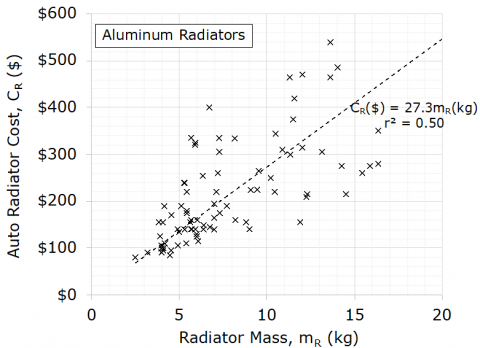## 8b.png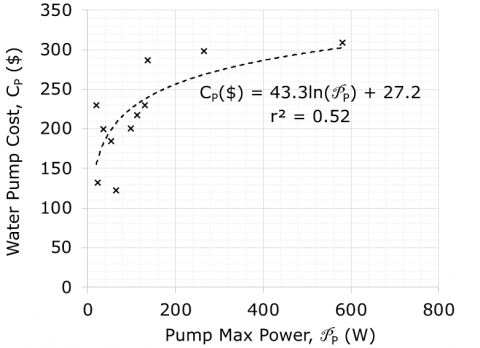## 8c.png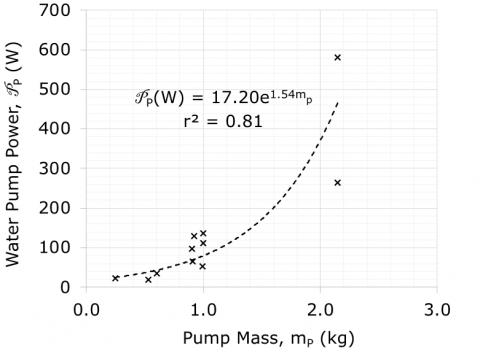## 8d.png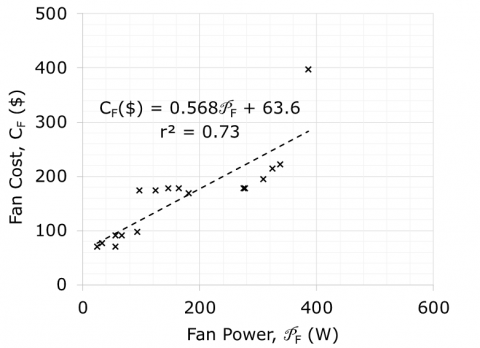## 8e.pngFigure 8. Relationship between cost, mass and power for the radiator system components

Table 2 shows the different input to the manufacturing and operation phases for the conventional and nanofluid-integrated radiator systems. It is apparent that for the same radiator effectiveness, the addition of just 1% of alumina to the radiator fluid (radiator system 2) results in a decrease in the size of the radiator and its system components (pump and fan). Even though the addition of nanoparticles to the coolant will cause a substantial increase in the fluid viscosity, the decrease in the size of the radiator outweighs the increase in viscosity (and pressure loss), and the net effect is a decrease in the pump size .

Energy consumption and environmental burdens associated with the economic demand from the different sectors of the U.S. economy are calculated for the manufacturing phase for both systems (Table 3). The environmental burdens consist of the equivalent CO2 emissions, water usage, and toxic releases. Since EIO-LCA model is based on a 2002 U.S. economy, the 2020 buying power of a U.S. dollar was deflated to reflect its buying power in 2002 .

The contribution of the radiator system to the vehicle fuel consumption is:

$F=M_{R} L F \frac{F E}{M_{V}} \frac{\Delta F}{\Delta M}$      (7)

where, F is the fuel consumed over the life of the radiator system, MR is the mass of the radiator system, LF is the life of the radiator system, FE is the car fuel economy factor, MV is the mass of the vehicle, and ∆F/∆M is the fuel consumption correlation with mass. The radiator life span was estimated to be 1.54x108 m. For CO, HC and NOx emissions, the contribution of the radiator system to the vehicle total air emissions, me, was :

$m_{e}=m_{e}^{\prime} F / F E$      (8)

where, $m_{e}^{\prime}$ is an emission factor equivalent to 11.1x10-6 kg.m-1 for CO, 0.57x10-6 kg.m-1 for HC and 1.3x10-6 kg.m-1 for NOx . For CO2, the emissions are estimated at 2.34x103 kg.m-3 of gasoline burnt. Table 4 and Figure 9 summarize the energy and environmental burdens.

It is shown that for the same radiator effectiveness, the addition of 1% of alumina to the radiator fluid reduces the energy consumption and global warming potential (GWP) of the manufacturing and operation phase by 28%, and water usage and total air releases by 29%. GWP is the amount of an equivalent CO2 gas that would warm the earth as much as that of one unit of other greenhouse gases, namely hydrocarbons (HC) and nitrogen oxide emissions (NOx). GWP has a weighing factor of 1 for 1 kg of CO2, a weighing factor of 25 for 1 kg of HC, and a weighing factor of 298 for 1 kg of NOx emissions. GWP for the greenhouse gas mixture was then calculated as follows:

$G W P=1 \times m_{C O_{2}}+25 \times m_{H C}+298 \times m_{N O_{x}}$      (9)

Table 2. Input to manufacturing and operation phase for the radiator systems

 System Component Conventional Radiator (System 1) Radiator-Embedded Nanofluid (System 2) Radiator Mass (kg) 4.29 3.15 Cost ($)$117.12 $86.00 Water Pump Power (W) 45 8.2 Mass (kg) 0.62 0.25 Cost ($) $192.03$118.38 Cooling Fan Power (W) 120 55.65 Mass (kg) 1.67 1.20 Cost ($)$131.76 $95.21 Fluids Coolant Mass (kg) 7.1 kg (6.4 L) 5.22 kg (3.9 L) AL2O3 Particles (kg) NA 0.17 Coolant Cost ($) $40.63$29.87 AL2O3 Particles Cost ($) NA$1.55 (5kg @ $48.7) Gasoline Oil Producer Price (56% Fuel Cost of$2.55) $17.78$12.98

Table 3. Energy and environmental burdens associated with the manufacturing phase

 System Component Economic Input Value ($) Water Usage (m3) Energy Consumed (MJ) Greenhouse Gas Emission, CO2,e (kg) Total Air Releases (10-3kg) System 1 Radiator 178 3.08 906 64.4 8.9 Water Pump 298 4.28 1140 75.6 16.4 Cooling Fan 200 2.85 845 52.5 12.5 Coolant 83.1 2.24 1200 83.0 11.8 Refineries 33.4 0.44 394 34.7 2.3 Total 793 12.89 4,485 310 52 System 2 Radiator 130 2.26 665 47.3 6.5 Water Pump 184 2.65 703 46.6 10.1 Cooling Fan 145 2.06 611 38.0 9.01 Coolant 61 1.65 883 61.0 8.6 Alumina 2.44 0.17 53 3.6 1.1 Refineries 24.4 0.32 287 25.3 1.7 Total 547 9.11 3,202 222 37 Table 4. Summary of energy and environmental burdens  Radiator System Water Usage (m3) Energy Consumed (MJ) GWP/Green-House CO2,e (kg) Total air Releases (kg) Manufacturing Phase System 1 12.89 4,485 310 52x10-3 System 2 9.11 3,202 222 37x10-3 Use Phase System 1 --- 1,668 373 --- System 2 --- 1,218 272 --- Manufacturing + Use Phase System 1 12.89 6,153 683 52x10-3 System 2 9.11 4,420 494 37x10-3 Sys 2/Sys 1 71% 72% 72% 71% ## 9.png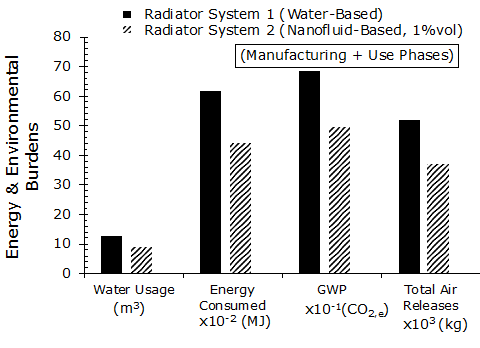Figure 9. Energy and environmental burdens 6. Conclusions A review was performed on the use of water-based alumina nanofluid as a heat transfer fluid. The paper compiled experimental data from various resources to examine the effect of the alumina nanoparticles concentration on the thermophysical properties and the bulk fluid Nusselt number for a radiator-type heat exchanger. Gathered data show the Nusselt number of the bulk fluid to increase by 50% with the introduction of 1% by volume of alumina nanoparticles to the base fluid. For the same automobile radiator effectiveness, this results in a 27% decrease in the radiator size, 60% decrease in the pump size and 28% decrease in the cooling fan size compared to a radiator using a conventional fluid. An economic input-output life cycle assessment study for the manufacturing and use life cycle phases was conducted on a 2017 Subaru Impreza radiator system, and on a resized radiator system having the same effectiveness but uses 1% by volume of alumina nanoparticles. The study shows a 28% reduction in the consumed energy and global warming potential, and a 29% reduction in the water usage and total air releases. Nomenclature  A area of interface between the hot and cold side of the heat exchanger, m2 C cost cp specific heat Cr ratio of minimum to maximum heat capacity rates D hydraulic diameter, m F fuel consumed over the life of the radiator system, m3 FE fuel economy of the vehicle, m3.m-1 f friction coefficient h heat transfer coefficient, W.m-2.K-1 L tubing length, m LF life of the radiator system, m m mass me vehicle total air emission, kg$m_{e}^{\prime}$emission factor, kg.m-1 MR total mass of the radiator system, kg MV mass of the vehicle, kg$\dot{m}$mass flow rate, kg.s-1 NTU number of transfer units Nu Nusselt number P Pressure, N.m-2$\wp$Power, W U radiator overall heat transfer coefficient, W.m-2.K-1 V Velocity, m.s-1 Greek symbols$\Delta$change$\varepsilon$heat exchanger effectiveness$\phi$concentration$\lambda$thermal conductivity$\mu$dynamic viscosity$\eta\$ overall efficiency of the radiator finned surface Subscripts 1 conventional radiator system 2 radiator utilizing a nanofluid coolant a air c radiator cold side f fluid F fan h radiator hot side np nanofluid o overall p pump R radiator v volume
References

 Farajollahi, B., Etemad, G., Hojjat, M. (2010). Heat transfer of nanofluids in a shell and tube heat exchanger. International Journal of Heat and Mass Transfer, 53(1-3): 12-17. https://doi.org/10.1016/j.ijheatmasstransfer.2009.10.019

 Rao, M., Sreeramulu, D., Naidu, D.A. (2016). Experimental investigation of heat transfer rate of nanofluids using a shell and tube heat exchanger. IOP Conference Series: Materials Science and Engineering, 149(1). https://doi.org/10.1088/1757-899X/149/1/012204

 Shahrul, I.M., Mahbubul, I.M., Saidur, R., Sabri, M. (2016). Experimental investigation of Al2O3-W, SiO2-W and ZnO-W nanofluids and their application in a shell and tube heat exchanger. International Journal of Heat and Mass Transfer, 97: 547-558. https://doi.org/10.1016/j.ijheatmasstransfer.2016.02.016

 Barzegarian, R., Aloueyan A., Yousefi, T. (2017). Thermal performance augmentation using water based Al2O3-gamma nanofluid in a horizontal shell and tube heat exchanger under forced circulation. International Communications in Heat and Mass Transfer, 86: 52-59. https://doi.org/10.1016/j.icheatmasstransfer.2017.05.021

 Akhtari, M., Haghshenasfard, M., Talaie, M.R. (2013). Numerical and experimental investigation of heat transfer of a-Al2O3/water nanofluid in double pipe and shell and tube heat exchangers. Numerical Heat Transfer, Part A: Applications, 63(12): 941-958. https://doi.org/10.1080/10407782.2013.772855

 Ahmed, M.S., Abdel Hady, M.R., Abdallah, G. (2018). Experimental investigation on the performance of chilled-water air conditioning unit using alumina nanofluids. Thermal Science and Engineering Progress, 5: 589-596. https://doi.org/10.1016/j.tsep.2017.07.002

 Anitha, S., Thomas, T., Parthiban, V., Pichumani, M. (2019). What dominates heat transfer performance of hybrid nanofluid in single pass shell and tube heat exchanger? Advanced Powder Technology, 30(12): 3107-3117. https://doi.org/10.1016/j.apt.2019.09.018

 Hojjat, M. (2020). Nanofluids as coolant in a shell and tube heat exchanger. Applied Mathematics and Computation, 365(3): 1-15. http://dx.doi.org/10.1016/j.amc.2019.124710

 Peyghambarzadeh, S.M., Hashemabadi, S.H., Jamnani, M.S., Hoseini, S.M. (2011). Improving the cooling performance of automobile radiator with Al2O3/water nanofluid. Applied Thermal Engineering, 31(10): 1833-1838. https://doi.org/10.1016/j.applthermaleng.2011.02.029

 Raei, B., Peyghambarzadeh, S.M., Asl, R.S. (2018). Experimental investigation on heat transfer and flow resistance of drag-reducing alumina nanofluid in a fin-and-tube heat exchanger. Applied Thermal Engineering, 144: 926-936. https://doi.org/10.1016/j.applthermaleng.2018.09.006

 Issa, R.J. (2016). Heat transfer investigation of aluminium oxide nanofluids in heat exchangers. European Scientific Journal, Special Edition, pp. 128-139.

 Ramalingam, S., Dhairiyasamy, R., Govindasamy, M. (2020). Assessment of heat transfer characteristics and system physiognomies using hybrid nanofluids in an automotive radiator. Chemical Engineering and Processing, 150: 1-12. https://doi.org/10.1016/j.cep.2020.107886

 Issa, R.J. (2021). A review on thermophysical properties and Nusselt number behavior of Al2O3 nanofluids in heat exchangers. Journal of Thermal Science, 30(2). https://doi.org/10.1007/s11630-021-1266-1

 Issa, R.J. (2016). Effect of nanoparticles size and concentration on thermal and rheological properties of AL2O3-water nanofluids. International Conference on Experimental and Numerical Flow and Heat Transfer (ENFHT’16), Prague, Czech Republic. https://doi.org/10.11159/enfht16.101

 Lee, J., Yoon, Y.J., Eaton, J.K., Goodson, K., Bai, S.J. (2014). Analysis of oxide (Al2O3, CuO, ZnO) and CNT nanoparticles disaggregation effect on the thermal conductivity and the viscosity of nanofluids. International Journal of Precision Engineering and Manufacturing, 15(4): 703-710. https://doi.org/10.1007/s12541-014-0390-1

 Chandrasekar, M., Suresh, S., Bose, A.C. (2010). Experimental investigations and theoretical determination of thermal conductivity and viscosity of AL2O3/water nanofluid. Experimental Thermal Fluid Science, 34(2): 210-216. https://doi.org/10.1016/j.expthermflusci.2009.10.022

 Mintsa, H.A., Roy, G., Nguyen, C.T., Doucet, D. (2009). New temperature dependent thermal conductivity data for water-based nanofluids. International Journal of Thermal Science, 48(2): 363-371. https://doi.org/10.1016/j.ijthermalsci.2008.03.009

 Das, S.K., Putra, N., Thiesen, P., Roetzel, W. (2003). Temperature dependence of thermal conductivity enhancement for nanofluids. Journal of Heat Transfer, 125(4): 567-574. https://doi.org/10.1115/1.1571080

 Ghanbarpour, M., Haghigi, E.B., Khodabandeh, R. (2014). Thermal properties and rheological behaviour of water based AL2O3 nanofluid as a heat transfer fluid. Experimental Thermal Fluid Science, 53: 227-235. https://doi.org/10.1016/j.expthermflusci.2013.12.013

 Duan, F. (2012). Thermal property measurement of AL2O3-water nanofluids. Smart Nanoparticles Technology, In Tech Europe, pp. 335-357. https://doi.org/10.5772/33830

 Eastman, J.A., Choi, U.S., Li, S., Thompson, L.J. (1997). Enhanced thermal conductivity through the development of nanofluids. Materials Research Society Symposium Proceedings, 457: 3-11. https://doi.org/10.1557/PROC-457-3

 Yiamsawasd, T., Dalkilic, S., Wongwises, S. (2012). Measurement of thermal conductivity of titania and alumina nanofluids. Thermochimica Acta, 545: 48-56. https://doi.org/10.1016/j.tca.2012.06.026

 Timofeeva, E.V., Gavrilov, A.N., McCloskey, J.M., Tolmachev, Y.V., Sprunt, S., Lopatina, L.M., Selinger, J.V. (2007). Thermal conductivity and particle agglomeration in alumina nanofluids: Experiment and theory. Physical Review E, 76: 1-16. https://doi.org/10.1103/PhysRevE.76.061203

 Murshed, S.M.S., Leong, K.C., Yang, C. (2008). Investigations of thermal conductivity and viscosity of nanofluids. Journal of Thermal Science, 47(5): 560-568. https://doi.org/10.1016/j.ijthermalsci.2007.05.004

 Nguyen, C.T., Desgranges, F., Galanis, N., Roy, G., Mare, T., Boucher, S., Mintsa, H.A. (2008). Viscosity data for AL2O3-water nanofluid hysteresis. International Journal of Thermal Science, 47(2): 103-111. https://doi.org/10.1016/j.ijthermalsci.2007.01.033

 Wu, Z., Wang, L., Sunden, B. (2013). Pressure drop and convective heat transfer of water and nanofluids in a double-pipe helical heat exchanger. Applied Thermal Engineering, 60(1-2): 266-274. https://doi.org/10.1016/j.applthermaleng.2013.06.051

 Darzi, A., Farhadi, M., Sedighi, K. (2013). Heat transfer and flow characteristics of Al2O3-water nanofluid in a double tube heat exchanger. International Communications in Heat and Mass Transfer, 47: 105-112. https://doi.org/10.1016/j.icheatmasstransfer.2013.06.003

 Akyurek, E.F., Gelis, K., Sahin, B., Manay, E. (2018). Experimental analysis for heat transfer of nanofluid with wire coil turbulators in a concentric tube heat exchanger. Results in Physics, 9: 376-389. https://doi.org/10.1016/j.rinp.2018.02.067

 Saidi, M.H., Mozafari, A.A., Sany, A.R.E., Neyestani, J. (2006). Experimental study of thermal performance and pressure drop in compact heat exchanger installed in automotive. Proceedings of ICES 2006, Aachen, Germany. https://doi.org/10.1115/ICES2006-1333

 Economic Input-Output Life Cycle Assessment Toolbox. http://www.eiolca.net, assessed on Feb. 9, 2021.

 Leontief, W. (1936). Quantitative input and output relations in the economic system of the United States. Review of Economics and Statistics, 18(3): 105-125. https://doi.org/10.2307/1927837

 Autozone, Inc. https://www.autozone.com/cooling-heating-and-climate-control/radiator, assessed on Feb. 20, 2021.

 SPAL Automotive. https://webstore.spalusa.com/en-us/product/0697/products/fans/4-fans, assessed on Fen 20, 2021.

 United States Inflator Calculator. https://www.usinflationcalculator.com, assessed on Feb 25, 2021.

 Keoleian, A., Spatari, S., Beal, R. (1998). Life Cycle Design of a Fuel Tank System. General Motors Demonstration Project, EPA 600/R-97-118.